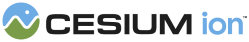#HermitePolynomialApproximation

An `InterpolationAlgorithm` for performing Hermite interpolation.

### Methods

#### static Cesium.HermitePolynomialApproximation.getRequiredDataPoints(degree, inputOrder) → number engine/Source/Core/HermitePolynomialApproximation.js 82

Given the desired degree, returns the number of data points required for interpolation.
Name Type Default Description
`degree` number The desired degree of interpolation.
`inputOrder` number `0` optional The order of the inputs (0 means just the data, 1 means the data and its derivative, etc).
##### Returns:
The number of required data points needed for the desired degree of interpolation.

#### static Cesium.HermitePolynomialApproximation.interpolate(x, xTable, yTable, yStride, inputOrder, outputOrder, result) → Array.<number> engine/Source/Core/HermitePolynomialApproximation.js 215

Interpolates values using Hermite Polynomial Approximation.
Name Type Description
`x` number The independent variable for which the dependent variables will be interpolated.
`xTable` Array.<number> The array of independent variables to use to interpolate. The values in this array must be in increasing order and the same value must not occur twice in the array.
`yTable` Array.<number> The array of dependent variables to use to interpolate. For a set of three dependent values (p,q,w) at time 1 and time 2 this should be as follows: {p1, q1, w1, p2, q2, w2}.
`yStride` number The number of dependent variable values in yTable corresponding to each independent variable value in xTable.
`inputOrder` number The number of derivatives supplied for input.
`outputOrder` number The number of derivatives desired for output.
`result` Array.<number> optional An existing array into which to store the result.
##### Returns:
The array of interpolated values, or the result parameter if one was provided.

#### static Cesium.HermitePolynomialApproximation.interpolateOrderZero(x, xTable, yTable, yStride, result) → Array.<number> engine/Source/Core/HermitePolynomialApproximation.js 116

Interpolates values using Hermite Polynomial Approximation.
Name Type Description
`x` number The independent variable for which the dependent variables will be interpolated.
`xTable` Array.<number> The array of independent variables to use to interpolate. The values in this array must be in increasing order and the same value must not occur twice in the array.
`yTable` Array.<number> The array of dependent variables to use to interpolate. For a set of three dependent values (p,q,w) at time 1 and time 2 this should be as follows: {p1, q1, w1, p2, q2, w2}.
`yStride` number The number of dependent variable values in yTable corresponding to each independent variable value in xTable.
`result` Array.<number> optional An existing array into which to store the result.
##### Returns:
The array of interpolated values, or the result parameter if one was provided.
Need help? The fastest way to get answers is from the community and team on the Cesium Forum.